Registration Required

## Please verify your email with the code we sent to your inbox

Имя пользователя/email, которое вы указали, уже зарегистрировано. Пожалуйста, выберите другое. Домен этого адреса электронной почты заблокирован. Пожалуйста, используйте другой домен Blocked domain

Отправить новый код

## Create your myAnalog password, and log in to access this article.

1. 8-16 символов
2. 3 из 4 должны содержать:
• Строчные буквы
• Заглавные буквы
• Цифры(0-9)
• Один или более из следующих символов:
@ # \$ % ^ & * - _ + = [ ] { } | \ : ' , ? / ` ~ " ( ) ; .

# If All Else Fails, Read This Article. Avoid Common Problems When Designing Amplifier Circuits

### Introduction

Modern operational amplifiers (op amps) and instrumentation amplifiers (in-amps) provide great benefits to the designer, compared with assemblies of discrete semiconductors. A great many clever, useful, and tempting circuit applications have been published. But all too often, in one’s haste to assemble a circuit, some very basic issue is overlooked that leads to the circuit not functioning as expected—or perhaps at all. This article will discuss a few of the most common application problems and suggest practical solutions.

### Missing DC Bias Current Return Path When AC-Coupled

One of the most common application problems encountered is the failure to provide a dc return path for bias current in ac-coupled operational- or instrumentation-amplifier circuits. In Figure 1, a capacitor is connected in series with the noninverting (+) input of an op amp to ac couple it, an easy way to block dc voltages that are associated with the input voltage (VIN). This should be especially useful in high-gain applications, where even a small dc voltage at an amplifier’s input can limit the dynamic range, or even result in output saturation. However, capacitively coupling into a high-impedance input, without providing a dc path for current flowing in the + input, will lead to trouble!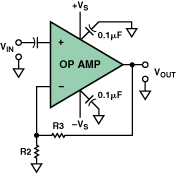Figure 1. A malfunctional ac-coupled op-amp circuit.

What actually happens is that the input bias currents will flow through the coupling capacitor, charging it, until the common-mode voltage rating of the amplifier’s input circuit is exceeded or the output is driven into limits. Depending on the polarity of the input bias current, the capacitor will charge up toward the positive supply voltage or down toward the negative supply. The bias voltage is amplified by the closed-loop dc gain of the amplifier.

This process can take a long time. For example, an amplifier with a field-effect-transistor (FET) input, having a 1-pA bias current, coupled via a 0.1-μF capacitor, will have a charging rate, I/C, of 10–12/10–7 = 10 μV/s, or 600 μV per minute. If the gain is 100, the output will drift at 0.06 V per minute. Thus, a casual lab test (using an ac-coupled scope) might not detect this problem, and the circuit will not fail until hours later. Obviously, it is very important to avoid this problem altogether.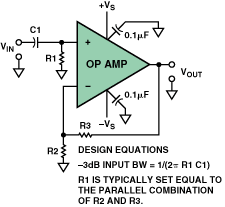Figure 2. Correct method for ac coupling an op-amp input for dual-supply operation.

Figure 2 shows a simple solution to this very common problem. Here, a resistor is connected between the op-amp input and ground to provide a path for the input bias current. To minimize offset voltages caused by input bias currents, which track one another when using bipolar op amps, R1 is usually set equal to the parallel combination of R2 and R3.

Note, however, that this resistor will always introduce some noise into the circuit, so there will be a trade-off between circuit input impedance, the size of the input coupling capacitor needed, and the Johnson noise added by the resistor. Typical resistor values are generally in the range from about 100,000 ohm to 1 Mohm.

A similar problem can affect an instrumentation amplifier circuit. Figure 3 shows in-amp circuits that are ac-coupled using two capacitors, without providing an input-bias-current return path. This problem is common with instrumentation amplifier circuits using both dual- (Figure 3a) and single (Figure 3b) power supplies.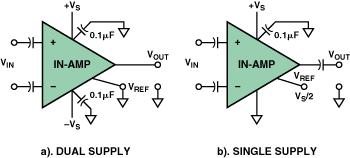Figure 3. Examples of nonfunctional ac-coupled in-amp circuits.

The problem can also occur with transformer coupling, as in Figure 4, if no dc return path to ground is provided in the transformer’s secondary circuit.Figure 4. A nonfunctional transformer-coupled in-amp circuit.

Simple solutions for these circuits are shown in Figure 5 and  Figure 6. Here, a high-value resistance (RA, RB) is added between each input and ground. This is a simple and practical solution for dual-supply in-amp circuits.Figure 5. A high-value resistor between each input and common supplies the necessary bias-current return path. a. Dual supply. b. Single supply.

The resistors provide a discharge path for input bias currents. In the dual-supply example of Figure 5a, both inputs are now referenced to ground. In the single-supply example of 5b, the inputs may be referenced either to ground (VCM tied to ground) or to a bias voltage, usually one-half the maximum input voltage range.

The same principle can be used for transformer-coupled inputs (Figure 6), unless the transformer secondary has a center tap, which can be grounded or connected to VCM.

In these circuits, there will be a small offset-voltage error due to mismatches between the resistors and/or the input bias currents. To minimize such errors, a third resistor, about 1/10th their value (but still large compared to the differential source resistance), can be connected between the two in-amp inputs (thus bridging both resistors).Figure 6. Correct method for transformer input coupling to an in-amp.

### Supplying Reference Voltages for In-Amps, Op Amps, and ADCs

Figure 7 shows a single-supply circuit where an in-amp is driving a single-ended analog-to-digital converter (ADC). The amplifier’s reference provides a bias voltage corresponding to zero differential input, and the ADC reference provides the scale factor. A simple RC low-pass antialiasing filter is often used between in-amp output and ADC input to reduce out-of-band noise. Often designers are tempted to use simple approaches such as resistance dividers to supply the in-amp and ADC reference voltages. This can lead to errors in the case of some in-amps.Figure 7. An in-amp drives an ADC in a typical single-supply circuit.

### Correctly Providing In-Amp Reference Voltage

A common assumption is that the in-amp’s reference-input terminal is at high impedance, since it’s an input. So a designer may be tempted to connect a high-impedance source, such as a resistive divider, to the reference pin of an in-amp. This can introduce serious errors with some types of instrumentation amplifiers (Figure 8).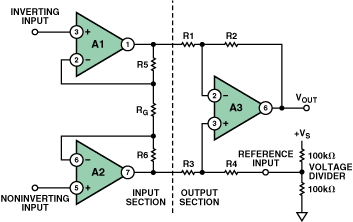Figure 8. Incorrect use of a simple voltage divider to directly drive the reference pin of a 3-op-amp instrumentation amplifier.

For example, a popular in-amp design configuration uses three op amps connected as above. The overall signal gain is

The gain for the reference input (if driven from low impedance) is unity. However, in the case shown, the in-amp has its reference pin tied directly to a simple voltage divider. This unbalances the symmetry of the subtractor circuit and the division ratio of the voltage divider. This would reduce the in-amp’s common-mode rejection and its gain accuracy. However, if R4 is accessible, so that its resistance value can be reduced by an amount equal to the resistance looking back into the paralleled legs of the voltage divider (50 kohm here), the circuit will behave as though a low-impedance voltage source equal to (in this example) one-half the supply voltage were applied to the original value of R4, and the subtractor’s accuracy would be maintained.

This approach cannot be used if the in-amp is provided as a closed single package (an IC). Another consideration is that the temperature coefficients of the resistors in the voltage divider should track those of R4 and the other resistors in the subtractor. Finally, the approach locks out the possibility of having the reference be adjustable. If, on the other hand, one attempts to use small resistor values in the voltage divider in an effort to make the added resistance negligible, this will increase power supply current consumption and increase the dissipation of the circuit. In any case, such “brute force” is not a good design approach.

Figure 9 shows a better solution, using a low-power op-amp buffer between the voltage divider and the in-amp’s reference input. This eliminates the impedance-matching and temperature-tracking problem and allows the reference to be easily adjustable.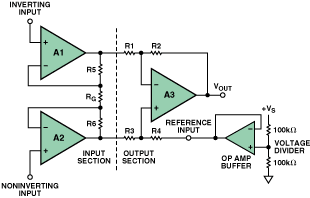Figure 9. Driving the reference pin of an in-amp from the low-impedance output of an op amp.

### Preserving Power-Supply Rejection (PSR) When Amplifiers Are Referenced from the Supply Rail Using Voltage Dividers

An often overlooked consideration is that any noise, transients, or drift of power-supply voltage, VS, fed in through the reference input will add directly to the output, attenuated only by the divider ratio. Practical solutions include bypassing and filtering, and perhaps even generating the reference voltage with a precision reference IC, such as the ADR121, instead of tapping off VS.

This consideration is important when designing circuits with both in-amps and op amps. Power-supply rejection techniques are used to isolate an amplifier from power supply hum, noise, and any transient voltage variations present on the power rails. This is important because many real-world circuits contain, connect to, or exist in environments that offer less-than-ideal supply voltage. Also, ac signals present on the supply lines can be fed back into the circuit, amplified, and under the right conditions, stimulate a parasitic oscillation.

Modern op amps and in-amps all provide substantial low-frequency power-supply rejection as part of their design. This is something that most engineers take for granted. Many modern op amps and in-amps have PSR specs of 80 dB to over 100 dB, reducing the effects of power-supply variations by a factor of 10,000 to 100,000. Even a fairly modest PSR spec of 40 dB isolates supply variations from the amplifier by a factor of 100. Nevertheless, high-frequency bypass capacitors (such as those in Figure 1 through Figure 7) are always desirable and often essential. In addition, when designers use a simple resistance divider on the supply rail and an op-amp buffer to supply a reference voltage for an in-amp, any variations in power-supply voltage are passed through this circuitry with little attenuation and add directly to the in-amp’s output level. So, unless low-pass filtering is provided, the normally excellent PSR of the IC is lost.

In Figure 10, a large capacitor has been added to the voltage divider to filter its output from power-supply variations and preserve PSR. The –3-dB pole of this filter is set by the parallel combination of R1/R2 and capacitor C1. The pole should be set approximately 10 times lower than the lowest frequency of concern.Figure 10. Decoupling the reference circuit to preserve PSR.

The “cookbook” values shown provide a –3-dB pole frequency of approximately 0.03 Hz. The small (0.01-μF) capacitor across R3 minimizes resistor noise.

The filter will take time to charge up. Using the cookbook values, the rise time at the reference input is several time constants (where T = R3Cf = 5 s), or about 10 to 15 seconds.

The circuit of Figure 11 offers a further refinement. Here, the op-amp buffer is operated as an active filter, which allows the use of much smaller capacitors for the same amount of power-supply decoupling. In addition, the active filter can be designed to provide a higher Q and thus give a quicker turn-on time.Figure 11. An op-amp buffer connected as an active filter drives the reference pin of an in-amp.

Test results: With the component values shown, and 12 V applied, a 6-V filtered reference voltage was provided to the in-amp. A 1-V p-p sine wave of varying frequency was used to modulate the 12-V supply, with the in-amp gain set to unity. Under these conditions, as frequency was decreased, no ac signal was visible on an oscilloscope, at VREF or at the in-amp output, until approximately 8 Hz. Measured supply range for this circuit was 4 V to greater than 25 V, with a low-level input signal applied to the in-amp. Circuit turn-on time was approximately 2 seconds.

### Decoupling Single-Supply Op-Amp Circuits

Finally, single-supply op-amp circuits require biasing of the input common-mode level to handle the positive and negative swings of ac signals. When this bias is provided from the power-supply rail, using voltage dividers, adequate decoupling is required to preserve PSR.

A common and incorrect practice is to use a 100-kohm/100-kohm resistor divider with a 0.1 μF bypass capacitor to supply VS/2 to the noninverting pin of the op amp. Using these values, power-supply decoupling is often inadequate, as the pole frequency is only 32 Hz. Circuit instability (“motor-boating”) often occurs, especially when driving inductive loads.

Figure 12 (noninverting) and Figure 13 (inverting) show circuits to accomplish VS/2 decoupled biasing for best results. In both cases, bias is provided at the noninverting input, feedback causes the inverting input to assume the same bias, and unity dc gain also biases the output to the same voltage. Coupling capacitor C1 rolls the low-frequency gain down toward unity from BW3.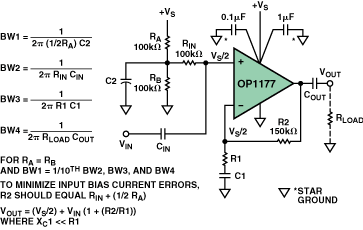Figure 12. A single-supply noninverting amplifier circuit, showing correct power-supply decoupling. Midband gain = 1 + R2/R1.

A good rule of thumb when using a 100 kohm/100 kohm voltage divider, as shown, is to use a C2 value of at least 10 μF for a 0.3-Hz –3-dB roll-off. A value of 100 μF (0.03-Hz pole) should be sufficient for practically all circuits.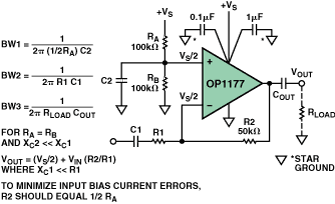Figure 13. Proper decoupling for a single-supply inverting-amplifier circuit. Midband gain = –R2/R1.